## Solubility Product

The solubility product is the equilibrium constant representing the maximum amount of solid that can be dissolved in aqueous solution.

### Learning Objectives

Calculate the solubility product constant of an aqueous solution.

### Key Takeaways

#### Key Points

• The general form of the solubility product constant (Ksp) for the equation: $\text{aA} (\text{s}) \rightleftharpoons \text{bB} (\text{aq}) + \text{cC} (\text{aq})$ is $\text{K}_{\text{sp}} = [\text{B}]^\text{b}[\text{C}]^\text{c}$.
• The smaller the solubility product, the lower the solubility. Solubility products are useful in predicting whether a precipitate will form under specified conditions.
• In an ICE table, the solubility of the solid is equal to the change (x) in the equilibrium calculation.

#### Key Terms

• precipitate: A solid that exits the liquid phase of a solution.
• solubility: The amount of a substance that will dissolve in a given amount of solvent to give a saturated solution under specified conditions.

The solubility product constant (Ksp) is the equilibrium constant for a solid that dissolves in an aqueous solution. All of the rules for determining equilibrium constants continue to apply. An equilibrium constant is the ratio of the concentration of the products of a reaction divided by the concentration of the reactants once the reaction has reached equilibrium. Consider this reaction:

$\text{AgCl} (\text{s}) \rightarrow \text{Ag}^+ (\text{aq}) + \text{Cl}^- (\text{aq})$

The equilibrium expression for the reaction is:

$\text{K}_{\text{eq}} = \frac{[\text{Ag}^+][\text{Cl}^-]}{[\text{AgCl}]}$

Because the AgCl is a solid, its concentration before and after the reaction is the same. The equilibrium equation can therefore be rearranged as:

$\text{K}_{\text{sp}} = [\text{Ag}^+][\text{Cl}^-]$

For substances in which the ions are not in a 1:1 ratio, the stoichiometric coefficients of the reaction become the exponents for the ions in the solubility-product expression:

$\text{PbCl}_2 \rightleftharpoons \text{Pb}^{2+} + 2\text{Cl}^- \text{ gives }\ \text{K}_{\text{sp}} = [\text{Pb}^{2+}][\text{Cl}^-]^2$

$\text{Ba}_3(\text{PO}_4)_2 \rightleftharpoons 3\text{Ba}^{2+} + {2\text{PO}_4}^{2-} \text{ gives }\ \text{K}_{\text{sp}}=[\text{Ba}^{2+}]^3[{\text{PO}_4}^{2-}]^2$

### Calculating the Solubility Product

At a certain temperature, the solubility of Fe(OH)2 in water is 7.7 x 10-6 mol/L (M).

Its Ksp can be calculated based on the equilibrium equation:

$\text{Fe}(\text{OH})_2 \rightleftharpoons \text{Fe}^{2+} + 2\text{OH}^-$

Therefore, the solubility product expression is:

$\text{K}_{\text{sp}} = [\text{Fe}^{2+}][\text{OH}^-]^2$

One mole of dissolved Fe(OH)2 produces one mole of Fe2+ and two moles of OH, so therefore:ICE table for the solubility of Fe(OH)2: The solubility of Fe(OH)2 is 7.7 x 10-6 M, this is equal to the value of the change (x) in the table.

$\text{K}_{\text{sp}} = \text{x}(2\text{x})^2$ where x = 7.7 x 10-6

$[\text{Fe}^{2+}] = 7.7 \times 10^{-6}$

$[\text{OH}^-] = 2 \times 7.7 \times 10^{-6} = 1.54 \times 10^{-5}$

$\text{K}_{\text{sp}} = [\text{Fe}^{2+}][\text{OH}^-]^2$

$\text{K}_{\text{sp}} = 7.7\times10^{-6} \times (1.54\times10^{-5})^2$

$\text{K}_{\text{sp}} = 1.83\times10^{-15}$

### Uses of Solubility Product

Solubility products are useful in predicting whether a precipitate will form under specified conditions. It is also helpful in choosing conditions under which two chemical substances in solution can be separated by fractional precipitation. The solubility product of a number of substances have been experimentally determined and can be used to predict solubility at a specified temperature.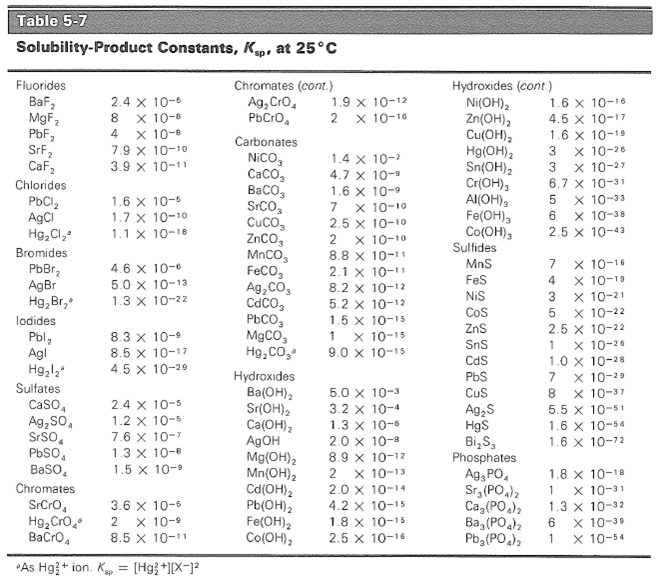Solubility product constants of common ions: The solubility product constants of a number of substances. Substances are grouped by anion and listed in the order of decreasing Ksp; anions are listed roughly in order of decreasing solubility.

## Molar Solubility and Relative Solubility

Molar solubility is the number of moles of a solute that can be dissolved per liter of solution before the solution becomes saturated.

### Learning Objectives

Calculate the molar solubility of a compound in water and the relative molar solubility of compounds

### Key Takeaways

#### Key Points

• A substance ‘s solubility product (Ksp) is the ratio of concentrations at equilibrium.
• The molar solubility of a compound can be calculated directly from its solubility product.
• Even if the solubility products of two compounds are similar, their molar solubilities can be very different.
• Scientists take advantage of the relative solubilities of compounds to separate or identify them.

#### Key Terms

• molar solubility: The number of moles of a substance (the solute) that can be dissolved per liter of solution before the solution becomes saturated.
• liter: A non-SI metric system unit of volume equal to 1 cubic decimeter (dm3), 1,000 cubic centimeters (cm3) or 1/1,000 cubic meter (m3)
• saturated solution: One in which the solvent can dissolve no more of a specific solute at a particular temperature.
• saturated: Containing all the solute that can normally be dissolved at a given temperature.

### Molar Solubility

A substance’s solubility product (Ksp) is the ratio of concentrations at equilibrium. Molar solubility, which is directly related to the solubility product, is the number of moles of the solute that can be dissolved per liter of solution before the solution becomes saturated. Once a solution is saturated, any additional solute precipitates out of the solution. The units are molarity (M), or mole liter-1 (mol/L).

### Calculating Molar Solubility

The relation between the molar solubility and the solubility product means that one can be used to find the other.

Example 1:

The Ksp for AgI is 8.5 x 10-17 at 25 °C. What is the molar solubility? (Let s = the solubility of the compound in water, usually defined as x in an ICE table.)

Solution:

The balanced equation for the reaction is:

$\text{AgI} (\text{s}) \leftrightarrow \text{Ag}^+ (\text{aq}) + \text{I}^- (\text{aq})$

The formula for Ksp is:

Ksp = [Ag+][I]

Ksp = s2 = 8.5 x 10-17

where s is the concentration of each ion at equilibrium. Now, solve for s:

s2 = 8.5 x 10-17

s = $\sqrt {8.5 \times 10^{-17} }$

s = 9.0 x 10-9 mol/L

The molar solubility of AgI is 9.0 x 10-9 mol/L.

Example 2:

The solubility products for cadmium carbonate (CdCO3) and silver carbonate (Ag2CO3) are almost exactly the same. Compare their molar solubilities in water at 25 °C.

Solution:

For cadmium carbonate:

$\text{CdCO}_3 (\text{s}) \leftrightarrow \text{Cd}^{2+} (\text{aq}) + \text{CO}_3\ ^{2+} (\text{aq})$

Ksp = [Cd2+][CO32-]

s2 = 5.2 x 10-12

s = 2.3 x 10-6 mol/L

For silver carbonate, the expression is slightly different. Since each mole of salt produces two moles of Ag+ ions, the value of Ag+ is 2s:

$\text{Ag}_2\text{CO}_3 (\text{s}) \leftrightarrow 2\text{Ag}^+ (\text{aq}) + \text{CO}_3^{2-} (\text{aq})$

Ksp = [Ag+]2[CO32-]

(2s)2s = 8.2 x 10-12

4s3 = 8.2 x 10-12

s = 1.3 x 10-4 mol/L

Although cadmium carbonate and silver carbonate have nearly the same solubility products, their solubilities in mol/L differ by a factor of 100. The solubility of silver carbonate is sensitive to the square of the metal-ion concentration because two silver ions per carbonate ion are necessary to build the solid crystal. Therefore, the form of the solubility product expression is different.

### Relative Solubility

If compounds have different solubilities, or relative solubilities, they can be separated. For example, in the process of extraction, scientists take something dissolved in one liquid and force it to become dissolved in another liquid. Caffeine must be extracted from coffee beans in order to be used in beverages such as soda. Typically, the caffeine is dissolved in carbon dioxide that has been heated to over 300 K and pressurized to 73 atm, making it a liquid. Then, the temperature is lowered (lowering the solubility of the caffeine in carbon dioxide), and water is added. The system is allowed to reach equilibrium. Since caffeine is more soluble in water than it is in carbon dioxide, the majority of it goes into the water.

A technique called paper chromatography also takes advantage of the relative solubilities of compounds. In paper chromatography, a small amount of the mixture is placed onto the paper approximately 1 cm from the edge. Then, the paper is suspended in a small quantity of the solvent in a closed container. As the solvent rises up the paper, the components in the mixture separate based on relative solubility. As the solvent nears the top, a mark is made to record the level, and the paper is removed and dried. Some components are colored and can be seen with the naked eye, but some need to be stained or irradiated with an ultraviolet lamp. The solute will always move at the same fraction of the distance of the solvent as long as temperature is held constant. The distance that the solute travels in a particular solvent can be used to identify the compound.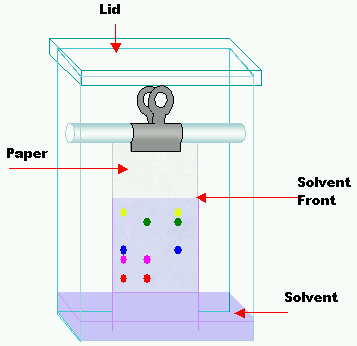Schematic of paper chromatography: Paper chromatography is an analytical chemistry technique for separating and identifying mixtures. Samples are spotted on the bottom of the paper. The end of the paper is placed in solvent. The solvent travels up the paper through capillary action. Mixtures are separated by their relative solubility in the solvent.

## Predicting Precipitation Reactions

A set of rules can be used to predict whether a combination of cations and anions in solution will recombine and precipitate.

### Learning Objectives

Use the rules of solubility to determine whether a precipitate forms when two solutes are mixed.

### Key Takeaways

#### Key Points

• Sometimes ions in solution react with each other to form a new substance that is insoluble (does not dissolve), called a precipitate.
• A set of rules can be used to predict whether salts will precipitate.
• The property of certain ions to precipitate can be used to isolate a particular ion from the solution.

#### Key Terms

• insoluble: That which cannot be dissolved.
• spectator ion: An ion that exists as a reactant and a product in a chemical reaction.
• precipitate: To come out of a liquid solution into solid form.
• precipitate: A solid that exits the liquid phase of a solution.
• anion: A negatively charged ion, as opposed to a cation.
• cation: A positively charged ion, as opposed to an anion.

### Precipitation Reactions

Sometimes ions in solution react with each other to form a new substance that precipitates; this reaction is called a precipitation reaction. A precipitate will form if any combination of cations and anions can become a solid.

### Rules of Precipitation

Note that soluble compounds will dissolve in water and insoluble compounds will not.

The following list summarizes the combinations that will form precipitates in solution:

• Nitrates (NO3): All are soluble.
• Potassium (K+), sodium (Na+), and ammonium (NH4+) salts: All are soluble.
• Chlorides (Cl), bromides (Br), and iodides (I): All are soluble except silver (Ag+), lead (II) (Pb2+), and mercury (II) (Hg2+) salts.
• Sulfates (SO42-): All are soluble except lead (II) (Pb2+), barium (Ba2+), and calcium (Ca2+).
• Carbonates (CO32-): All are insoluble except those of potassium (K+), sodium (Na+), and ammonium (NH4+).
• Compounds with fluorine: All are soluble except magnesium (Mg2+), calcium (Ca2+), strontium (Sr2+), barium (Ba2+), and lead(II) (Pb2+).
• Perchlorates (ClO4) and acetates (C2H3O2): All are soluble.
• Metal hydroxides (OH) and oxides (O2-): Most are insoluble.
• Salts of phosphates (PO43-), oxalates (C2O42-), chromates (CrO42-), and sulfides (S2-): Generally insoluble.

### Example of Precipitation Reaction

Imagine you have two test tubes and three solutions: copper (II) chloride (CuCl2) solution, sodium carbonate (Na2CO3) solution, and sodium sulfate (Na2SO4) solution. You put 5 mL of copper (II) chloride into tubes 1 and 2. You add 5 mL of sodium carbonate in tube 1. A precipitate, which looks like light blue specks of dust, forms. You carefully add the sodium sulphate solution into tube 2. No precipitate forms. The solution stays light blue. What happened?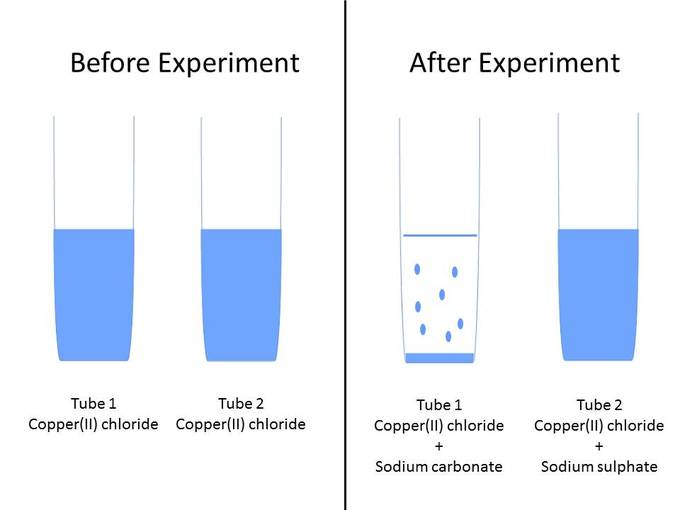Precipitation experiment: Example set up for precipitation experiment.

For reaction 1, you have the following ions in your solution: Cu2+, Cl, Na+, and CO32-. The product that forms may be insoluble, in which case a precipitate will form, or soluble, in which case the solution will be clear. Let’s see how the ions in this example could have combined with each other:

$\text{Cu}^{2+} + \text{CO}_3\ ^{2-} \rightarrow \text{CuCO}_3$

$\text{Cu}^{2+} + 2\text{Cl}^- \rightarrow \text{CuCl}_2$

$\text{Na}^+ + \text{Cl}^- \rightarrow \text{NaCl}$

$2\text{Na}^+ + \text{CO}_3\ ^{2-} \rightarrow \text{Na}_2\text{CO}_3$

You can automatically exclude the reactions where sodium carbonate and copper (II) chloride are the products because these were the initial reactants. The balanced chemical equation is:

${2\text{Na}^+} + {\text{Cu}^{2+}} +{\text{CO}_3\ ^{2-}} + {\text{Cl}^-} \rightarrow {\text{CuCO}_3} + {2\text{Na}^+} + {2\text{Cl}^-}$

You know that sodium chloride (NaCl) is soluble in water, so the remaining product (copper carbonate) must be the one that is insoluble. According to the rules of precipitation, the only soluble carbonates (CO32-) are potassium (K+), sodium (Na+), and ammonium (NH4+). Therefore Na2CO3 will remain in solution, but CuCO3 will precipitate out.

Notice how the sodium and chloride ions remain unchanged during the reaction. They are called spectator ions. They can be removed from the equation yielding the overall precipitation reaction:

$\text{Cu}^{2+} + \text{CO}_3\ ^{2-} \rightarrow \text{CuCO}_3$

Reaction 2 has Cu2+, Cl, Na+, and SO42- in solution. The possible combinations of the ions are as follows:

$\text{Cu}^{2+} + \text{SO}_4\ ^{2-} \rightarrow \text{CuSO}_4$

$\text{Na}^+ + \text{Cl}^- \rightarrow \text{NaCl}$

$\text{Cu}^2+ 2\text{Cl}^- \rightarrow \text{CuCl}_2$

$2\text{Na}^+ + \text{SO}_4\ ^{2-} \rightarrow \text{Na}_2\text{SO}_4$

Once again, the reactions where sodium sulfate and copper (II) chloride are the products can be excluded, since they were the initial reactants. The balanced chemical equation is:

${2\text{Na}^+} + {\text{SO}_4\ ^{2-}} + {\text{Cu}^{2+}} + {2\text{Cl}^ -} \rightarrow {2\text{Na}^+} + {\text{SO}_4\ ^{2-}} + {\text{Cu}^{2+}} + {2\text{Cl}^-}$

If we determine which of these salts are soluble and which are insoluble according to the rules, we see that most chlorides and most sulfates are soluble. This is why no precipitate forms in this second reaction. Even when the ions recombine, they immediately separate and go back into solution.

### Application of Precipitation Reactions

Precipitation reactions are often used to isolate a particular ion from the solution. The process allows for selective removal of ions through properties of solubility. The property is used to separate ions in a method called fractional precipitation.

## The Effect of pH on Solubility

By changing the pH of the solution, you can change the charge state of the solute.

### Learning Objectives

Describe the effect of pH on the solubility of a particular molecule.

### Key Takeaways

#### Key Points

• If the pH of the solution is such that a particular molecule carries no net electric charge, the solute often has minimal solubility and precipitates out of the solution.
• The pH at which the net charge of the solute is neutral is called the isoelectric point.
• At a pH below a molecule’s pI, that molecule will carry a net positive charge; at a pH above its pI, the molecule will carry a net negative charge.
• Isoelectric focusing can be used to separate different compounds in a mixture, particularly proteins.

#### Key Terms

• cathode: The electrode of an electrochemical cell at which reduction occurs.
• anode: The electrode of an electrochemical cell at which oxidation occurs.
• isoelectric point: The pH at which a particular molecule or surface carries no net electrical charge

### Solubility is Affected by pH

The pH of an aqueous solution can affect the solubility of the solute. By changing the pH of the solution, you can change the charge state of the solute. If the pH of the solution is such that a particular molecule carries no net electric charge, the solute often has minimal solubility and precipitates out of the solution. The pH at which the net charge is neutral is called the isoelectric point, or pI (sometimes abbreviated to IEP).

### Using pI to Separate Compounds

As an example, proteins are composed of linked compounds called amino acids. Amino acids all contain the same backbone, which has both an acidic and a basic group. Each amino acid also has a functional group attached to the backbone. These functional groups can be positive, negative, neutral, or polar in nature. The backbone and functional groups give a protein its overall charge. At a pH below the protein’s pI, a protein will carry a net positive charge; above its pI, it will carry a net negative charge. Proteins can therefore be separated according to their isoelectric point.Amino acid backbone as a zwitterion: The backbone of all amino acids contains both acidic (carboxylic acid fragment) and basic (amine fragment) centers. The isomer on the right is a zwitterion. The R indicates where the amino acid specific group is attached.

In a method called isoelectric focusing, proteins are run through a gel that has a pH gradient. The gels are set in a buffer in a container with a negatively charged electrode ( cathode ) on one end and a positively charged electrode (anode) on the other. When the proteins are added to the solution and current is applied, they migrate toward the electrode with the opposite charge (remember that opposites attract).

For example, a protein that is in a pH region below its isoelectric point will be positively charged and so will migrate towards the cathode (negative charge). As it migrates through a gradient of increasing pH, however, the protein’s overall charge will decrease until the protein reaches the pH region that corresponds to its pI. At this point, it has no net charge, and so it stops moving in the gel. The proteins become focused into sharp stationary bands with each protein positioned at a point in the pH gradient corresponding to its pI. This technique is capable of extremely high resolution and can separate proteins that differ by as little as a single charge.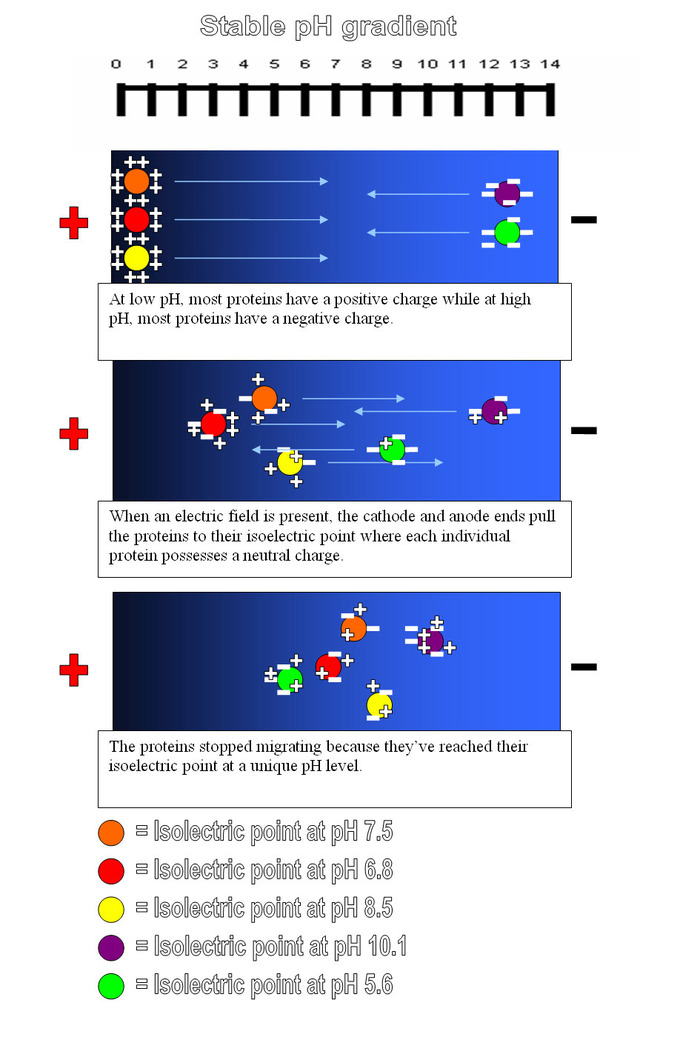Isoelectric focusing: Overview of an isoelectric focusing experiment to separate proteins based on the differences in their solubilities at different pH values.

## Ion Separation by Fractional Precipitation

Fractional precipitation can be used to determine which ions are present in a solution by taking advantage of their different solubilities.

### Learning Objectives

Calculate the amount of an assay that will be required to ensure precipitation.

### Key Takeaways

#### Key Points

• Fractional precipitation tests for certain ions using color change, solid formation, and other visible changes.
• Chlorides, bromides, and iodides can be detected by adding a small amount of silver nitrate solution.
• Sulfates can be detected with barium chloride solution.
• Carbonates can be detected with a small amount of acid.

#### Key Terms

• precipitation: A reaction that leads to the formation of a heavier solid in a lighter liquid; the precipitate so formed at the bottom of the container.
• distilled water: Water that has been purified by distillation.

### Fractional Precipitation

Chemists are often presented with a sample and are asked to figure out what chemicals are in the compound or solution. One of the methods that can be applied to the solution is fractional precipitation. Fractional precipitation takes advantage of the different solubilities of ions to determine which ions are present in a solution. Testing for certain ions is accomplished using color change, solid formation, and other visible changes.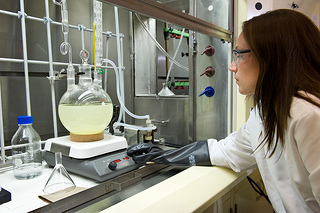Chemist analyzing unknown sample: U.S. Army scientists analyze unknown samples to determine whether hazardous chemical or biological warfare agents are present. Samples come from around the world.

### Example 1: Chloride or Carbonate Identification

Prepare a solution of the unknown salt using distilled water and add a small amount of silver nitrate (AgNO3) solution. If a white precipitate forms, the salt is either a chloride or a carbonate. Why?

If there is chlorine in the solution, the balanced reaction would be:

$\text{Cl}^{-} + \text{Ag}^{+} + \text{NO}_3^{-} \rightarrow \text{AgCl} + \text{NO}_3^{-}$

Silver chloride (AgCl) is not soluble and forms a white precipitate.

If there is carbonate in the solution, the balanced equation for the reaction would be:

${\text{CO}_{3}}^{2-} +2\text{Ag}^+ +2\text{NO}_3^{-} \rightarrow \text{Ag}_2\text{CO}_3 + 2\text{NO}_3^-$

Silver carbonate (Ag2CO3) is also not soluble in water.

So how does one tell if the precipitate is a carbonate or a chloride? Treat the precipitate with a small amount of concentrated nitric acid (HNO3). If the precipitate remains unchanged, then the salt is a chloride. If carbon dioxide is formed and the precipitate disappears, the salt is a carbonate. Here are the balanced equations:

$\text{AgCl} (\text{s}) + \text{HNO}_3 (\text{l}) \rightarrow$ (no reaction; precipitate is unchanged)

$\text{Ag}_2\text{CO}_3 (\text{s}) + 2\text{HNO}_3 (\text{l}) \rightarrow 2\text{Ag}^+ (\text{aq}) + 2\text{NO}_3^{-} (\text{aq}) + \text{H}_2\text{O} (\text{l}) + \text{CO}_2 (\text{g})$

In the above reaction, the silver carbonate is used up and the precipitate will disappear.

### Example 2: Bromide or Iodide Identification

Bromides and iodides also form precipitates when they are reacted with silver nitrate, but both precipitates are pale yellow. To determine whether the precipitate is a bromide or iodide, use water with chlorine dissolved in it and the organic solvent carbon tetrachloride (CCl4). Chlorine water frees the bromine and iodine atoms as a gas. The bromine gas turns the CCl4 a reddish brown:

$2\text{Br}^{-} + \text{Cl}_2 \rightarrow 2\text{Cl}^{-} + \text{Br}_2$

$\text{Br}_2 + \text{CCl}_4 \rightarrow$ (reddish brown colored solution)

The iodine gas turns the CCl4 purple:

$2\text{I}^{-} + \text{Cl}_2 \rightarrow 2\text{Cl}^{-} + \text{I}_2$

$\text{I}_2 + \text{CCl}_4 \rightarrow$ (purple colored solution)

### Using Ksp in Fractional Precipitation

Solubility products (Ksp) can be used to complement the above methods. Knowing about the solubility of the ions can help determine how much of the assay compound needs to be added to guarantee precipitation.

For example, consider a solution the has 0.01 M barium chloride (BaCl2) and 0.02 M strontium chloride (SrCl2). Can either Ba2+ or Sr2+ be precipitated selectively with concentrated sodium sulfate (Na2SO4) solution? Which ion will precipitate first?

To find the solution, consider that the barium sulfate (BaSO4) solubility is given by:

$\text{K}_{\text{sp}} = [\text{Ba}^{2+}][{\text{SO}_{4}}^{2-}] = 1.5 \times 10^{-9}$

Rearrange the equation to solve for the concentration of SO42-. This will give you the value that the sulfate ion concentration must reach to precipitate BaSO4.

$[\text{SO}_4\ ^{2-}] = \frac{\text{K}_{\text{sp}}}{[\text{Ba}^{2+}]}$

With 0.01 M of Ba2+, barium sulfate will not precipitate until the sulfate ion concentration increases to:

$[\text{SO}_4\ ^{2-}] = \frac{1.5 \times 10^{-9}}{0.01}$

[SO42-] = 1.5 x 10-7

The Ksp for strontium sulfate (SrSO4) is 7.6 x 10-7.Strontium sulfate will precipitate when the sulfate concentration is:

$\text{K}_{\text{sp}} = [\text{Sr}^{2+}][{\text{SO}_{4}}^{2-}] = 7.6 \times 10^{-7}$

$[\text{SO}_4\ ^{2-}] = \frac{7.6 \times10^{-7}}{0.02}$

[SO42-] = 3.8 x 10-5

If the sulfate ion is slowly added to the container containing both the Ba2+ and Sr2+ ions, the barium sulfate will precipitate first. This is because it requires a lower concentration of the sulfate ion (1.5 x 10-7 < 3.8 x 10-5) to precipitate.

## Effect of a Common Ion on Solubility

Adding a common ion decreases the solubility of a solute, causing it to precipitate.

### Learning Objectives

Calculate the molar solubility of a compound in solution containing a common ion.

### Key Takeaways

#### Key Points

• Adding a common ion decreases the solubility of a solute.
• The common-ion effect can be used to separate compounds or remove impurities from a mixture.
• Different common ions have different effects on the solubility of a solute based on the stoichiometry of the balanced equation.

#### Key Terms

• precipitate: A solid that exits the liquid phase of a solution.
• precipitate: To come out of a liquid solution into solid form.
• limestone: An abundant rock of marine and fresh-water sediments; primarily composed of calcite (CaCO₃); it occurs in a variety of forms, both crystalline and amorphous

### Adding a Common Ion

If you have a solution and solute in equilibrium, adding a common ion (an ion that is common with the dissolving solid) decreases the solubility of the solute. This is because Le Chatelier’s principle states the reaction will shift toward the left (toward the reactants ) to relieve the stress of the excess product. When equilibrium is shifted toward the reactants, the solute precipitates.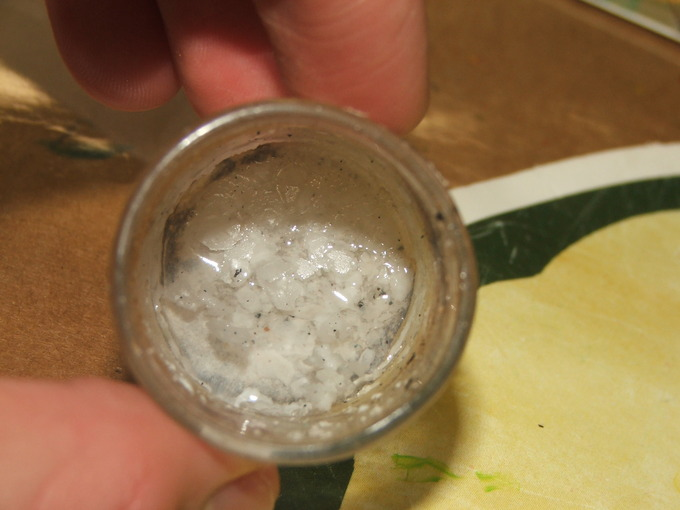Lithium hydroxide with carbonate growths: Lithium hydroxide forms less-soluble lithium carbonate, which precipitates because of the common ion effect.

Scientists take advantage of this property when purifying water. In areas where water sources are high in chalk or limestone, drinking water contains excess calcium carbonate CaCO3. In the water treatment process, sodium carbonate salt is added to precipitate the calcium carbonate. The very pure and finely divided precipitate of calcium carbonate that is generated is used in the manufacture of toothpaste.

### Example:

What is the solubility at 25°C of calcium fluoride (CaF2): (a) in pure water; (b) in 0.10 M calcium chloride (CaCl2); and (c) in 0.10 M sodium fluoride (NaF)?

Solution:

$\text{CaF}_2 \leftrightarrow \text{Ca}^{2+} + 2\text{F}^-$

(a) If the solubility in pure water is s, then

[Ca2+] = s and [F] = 2s

$\text{K}_{\text{sp}} = {[\text{Ca}^{2+}]}{[\text{F}^-]}^2$

Ksp = s(2s)2 = 4s3 = 3.9 x 10-11

s = 2.1 x 10-4 M

(b) Here the calcium ion concentration is the sum of the concentrations of calcium ions from the 0.10 M calcium chloride and from the calcium fluoride whose solubility we are seeking:

[Ca2+] = 0.10 + s

[F] = 2s

Ksp = (0.10 + s)(2s)2 = 3.9 x 10-11

Can we simplify this equation? With such a small solubility product for CaF2, you can predict its solubility << 0.10 moles per liter. If our prediction is valid, we can simplify the solubility-product equation:

Ksp = 0.10 (2s)2 = 0.40s2 = 3.9 x 10-11

s2 = $\frac{3.90 \times 10^{-11}}{0.40}$ = 9.75 x 10-11

s = 9.9 x 10-6 M

If we go back and compare, only 4.7 percent as much CaF2 will dissolve in 0.10 M CaCl2 as in pure water:

$\frac{(9.9 \times 10^{-6})}{2.1 \times 10^{-4}}$ x 100 = 4.7%

Therefore, the approximation that s is small compared to 0.10 M was reasonable.

(c) In 0.10 M NaF:

[Ca2+] = s

[F] = 0.10 + 2s

since fluoride ions are in NaF as well as in CaF2.

The solubility-product equation is:

Ksp = s(2s + 0.10)2 = 3.9 x 10-11

Again, the equation can be simplified. The 2s term is << 0.10 moles per liter, and therefore:

Ksp = s(0.10)2 = 3.9 x 10-11

s = 3.9 x 10-9 M

This approximation is also valid, since only 0.0019 percent as much CaF2 will dissolve in 0.10 M NaF as in pure water. Fluoride is more effective than calcium as a common ion because it has a second-power effect on the solubility equilibrium.